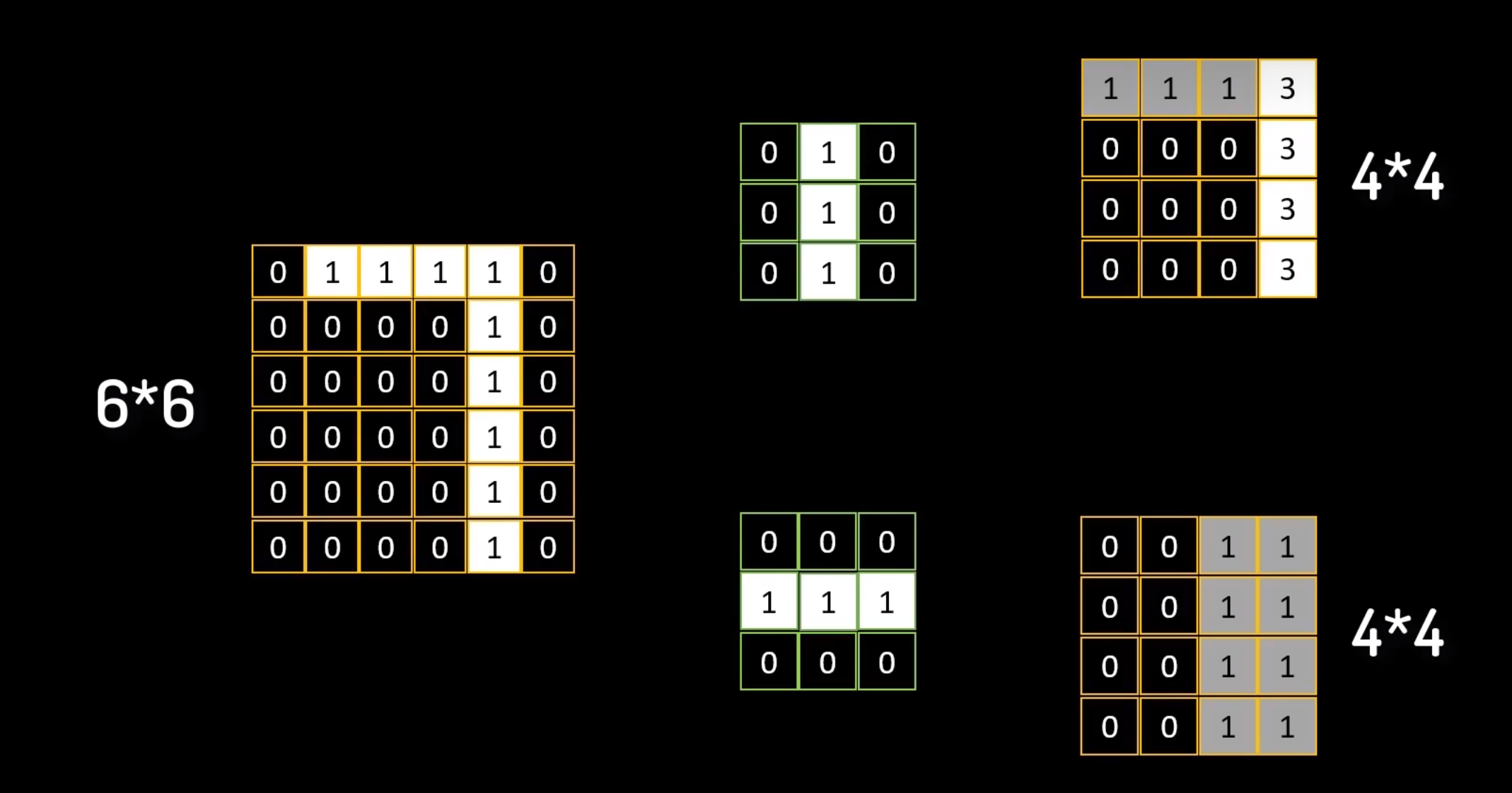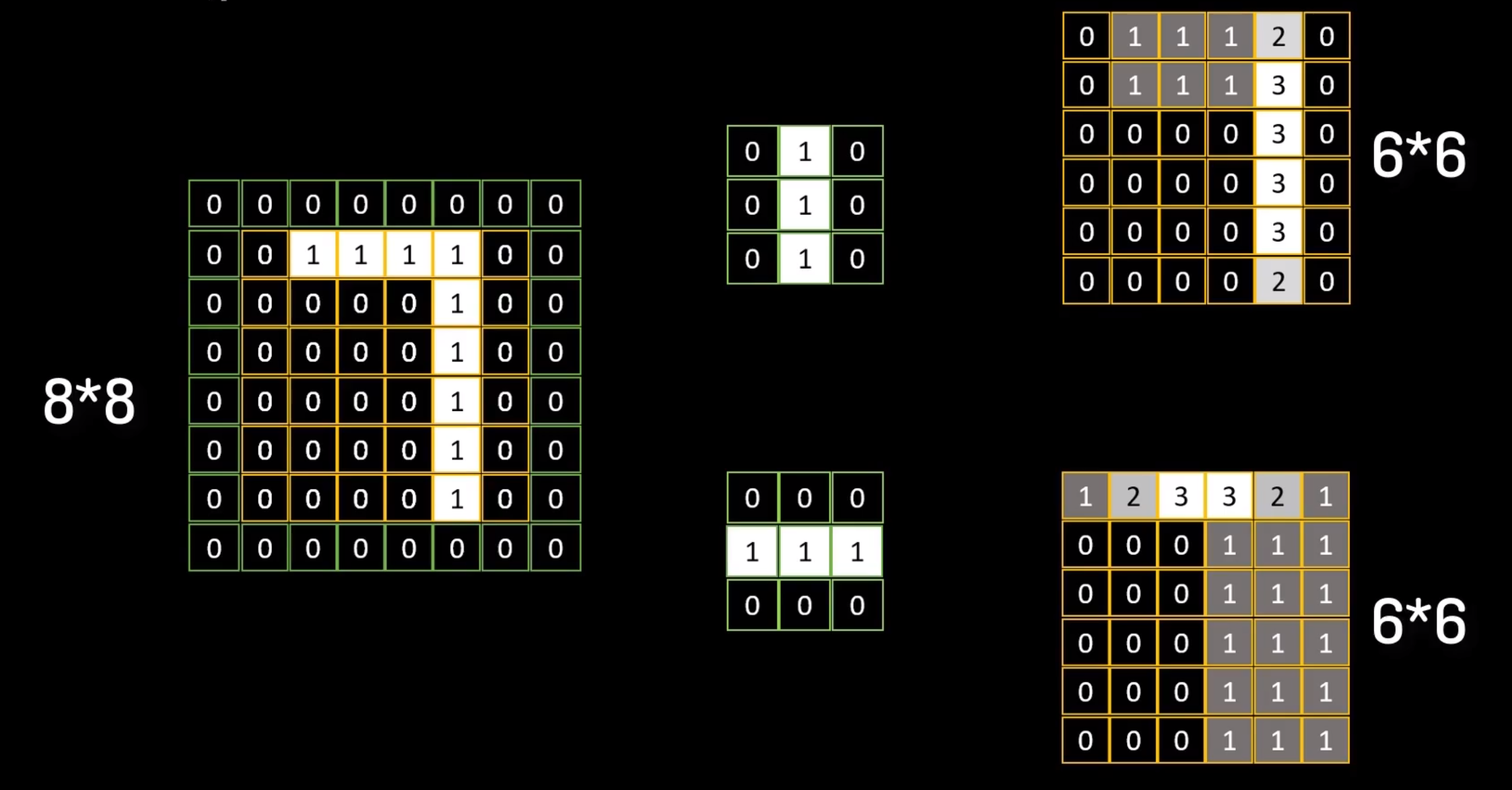## 【深度学习_TensorFlow】卷积神经网络（CNN）

news/2023/12/3 11:16:12/文章来源:https://blog.csdn.net/m0_73222051/article/details/133419831

## 写在前面

1. 先根据视频了解卷积和卷积神经网络的整体框架

2. 接着了解卷积神经网络构建过程中的一些重要操作，包括内积、填充、池化。

3. 然后介绍卷积层如何实现。

4. 最后用卷积神经网络的开山之作（LeNet-5）来进行上手练习。

### 一、初识卷积

#### 1. 卷积优势#### 2. 局部相关#### 3. 填充与池化### 二、卷积神经网络Layer (type)                Output Shape              Param #
=================================================================conv2d (Conv2D)             (None, 26, 26, 6)         60        max_pooling2d (MaxPooling2D  (None, 13, 13, 6)        0         )                                                               conv2d_1 (Conv2D)           (None, 11, 11, 16)        880       max_pooling2d_1 (MaxPooling  (None, 5, 5, 16)         0         2D)                                                             flatten (Flatten)           (None, 400)               0         dense (Dense)               (None, 120)               48120     dense_1 (Dense)             (None, 84)                10164     dense_2 (Dense)             (None, 10)                850       =================================================================

1. 经过一个卷积层（convolution），得到[b, 26, 26, 6]形状的张量，由于代码没有填充，故张量尺寸会缩减，具体的计算公式如下：其中(b, h’, w’, c)的张量，卷积核的数量c，卷积核的大小k，步长s， p h p_h 表示上下填充行数（只考虑两端相等）， p w p_w 表示左右填充列数（只考虑相等情况），在这里

h ′ = [ h + 2 ⋅ p h − k s ] + 1 h'=\left[\frac{h+2\cdot p_h-k}{s}\right]+1

h ′ = [ h + 2 ⋅ p w − k s ] + 1 h'=\left[\frac{h+2\cdot p_w-k}{s}\right]+1

h ′ = [ 28 + 2 ∗ 0 − 3 1 ] + 1 = ⌊ 25 ⌋ + 1 = 26 w ′ = [ 28 + 2 ∗ 0 − 3 1 ] + 1 = ⌊ 25 ⌋ + 1 = 26 \begin{aligned}h^{\prime} & =\left[\frac{28+2*0-3}{1}\right]+1=\lfloor25\rfloor+1=26\\ & \\ w^{\prime} & =\left[\frac{28+2*0-3}{1}\right]+1=\lfloor25\rfloor+1=26\end{aligned}

1. 经过一个向下采样层，张量尺寸缩小为[b, 13, 13, 6]，这里我们使用池化层来实现；

2. 经过第二个卷积层，得到[b, 11, 11, 16]的张量；

3. 经过第二个向下采样层，张量尺寸缩小为[b, 5, 5, 16]，这里我们使用池化层实现；

4. 将张量打平为[b, 400]，之后进入三个节点为 120、84、10 的全连接层将结果矩阵输出

### 三、卷积实现

#### 1. 自定义权值

tf.nn.conv2d(input, kernel, strides=1, padding=[[0, 0], [0, 0], [0, 0], [0, 0]])

input：输入数据图像，例如[100, 28, 28, 3]表示 100 张 28×28的三通道图像。

kernel：卷积核，例如[3, 3, 3, 4] 表示4个 3×3 的三通道的卷积核

strides：表示步长

padding：表示填充，填充的具体数量由tensorflow自动计算并完成

input = tf.random.normal([100, 28, 28, 3])
w = tf.random.normal([3, 3, 4, 3])out = tf.nn.conv2d(x, w, strides=1, padding=[[0, 0], [0, 0], [0, 0], [0, 0]])


#### 2. 卷积层的实现

# strides=1, padding='same'，可得到输入输出同大小的卷积层，具体填充操作自动完成
layers = layers.Conv2D(4, kernel_size=3, strides=1, padding='SAME')


### 四、leNet-5实战

# import tensorflow as tf
# from tensorflow.keras import datasets, layers, optimizers, Sequential, metrics, regularizers
# from tensorflow.keras.callbacks import EarlyStopping
# # 处理每一张图像
# def preprocess(x, y):
#
#     x = tf.cast(x, dtype=tf.float32) / 255.
#     y = tf.one_hot(y, depth=10)
#
#     return x, y
#
# # 数据集读取
# (x, y), (x_test, y_test) = datasets.mnist.load_data()
#
# # 交叉验证切分，防止过拟合的有效手段
# idx = tf.range(60000)  # 按顺序生成索引0~59999
# idx = tf.random.shuffle(idx)  # 将索引打乱
# x_train, y_train = tf.gather(x, idx[:50000]), tf.gather(y, idx[:50000])
# x_val, y_val = tf.gather(x, idx[-10000:]), tf.gather(y, idx[-10000:])
#
# # 训练集
# train_db = tf.data.Dataset.from_tensor_slices((x_train, y_train))
# train_db = train_db.map(preprocess).shuffle(10000).batch(128).repeat(5)  # 这里粗暴的重复数据增加数据集，repeat改小训练时间缩短
#
# # 验证集
# val_db = tf.data.Dataset.from_tensor_slices((x_val, y_val))
# val_db = val_db.map(preprocess).shuffle(10000).batch(128)
#
# # 测试集
# test_db = tf.data.Dataset.from_tensor_slices((x_test, y_test))
# test_db = test_db.map(preprocess).shuffle(10000).batch(128)
#
# # 定义早停法回调函数
# early_stopping = EarlyStopping(monitor='val_loss',  # 监视验证集loss
#                                patience=3,  # 当验证集loss在2个epoch内都没有改善则停止训练
#                                mode='min',  # 监测loss时一般设置为min，监测准确值时一般设置为max
#                                verbose=1,  # 检测值改善时打印一条信息
#                                restore_best_weights=True  # 将权重恢复到最好的一个epoch
#                                )
#
# # 网络构建
# # 正则化：在模型损失函数中加入权重参数的L2范数作为惩罚项,力度由0.001控制。
# network = Sequential([
#                       layers.Reshape(target_shape=(28, 28, 1), input_shape=(28, 28)),  # 这里指定了层，就不需要在process里进行reshape了，也不需要built构建参数了
#                       layers.Conv2D(6, kernel_size=3, strides=1),  # 6个3×3的卷积核，步长设置为1
#                       layers.MaxPooling2D(pool_size=(2, 2), strides=2),  # 高宽减半的池化层
#                       layers.Conv2D(16, kernel_size=3, strides=1),  # 第二个卷积层，16个3×3的卷积核
#                       layers.MaxPooling2D(pool_size=(2, 2), strides=2),  # 高宽减半的池化层
#                       layers.Flatten(),  # 打平，方便连接全连接层
#                       layers.Dense(120, kernel_regularizer=regularizers.l2(0.001), activation='relu'),
#                       layers.Dense(84, kernel_regularizer=regularizers.l2(0.001), activation='relu'),
#                       layers.Dense(10)
#                     ])
#
# # 参数构建
# # network.build(input_shape=(None, 28, 28, 1))  # 由于第一层已经指定好大小了，所以这里不构建也可以
# # 模型展示
# network.summary()
# # 模型装配
#                 loss=tf.losses.CategoricalCrossentropy(from_logits=True),
#                 metrics=['accuracy'])
# # 模型训练，这里控制训练次数
# network.fit(train_db, epochs=50,
#             validation_data=val_db, validation_steps=10,
#             callbacks=[early_stopping])
# # 模型评估
# print('模型评估：')
# network.evaluate(test_db)# ~~~~~~~~~~~~~~~~~~~~~~~~~~~~~~~~~~~~~~~~~~~~# 这两段代码框架一致，只是在不同位置进行了数据处理，注意辨别
import tensorflow as tf
from tensorflow.keras import datasets, layers, optimizers, Sequential, regularizers
from tensorflow.keras.callbacks import EarlyStopping# 处理每一张图像
def preprocess(x, y):x = tf.cast(x, dtype=tf.float32) / 255.x = tf.reshape(x, [28, 28, 1])y = tf.cast(y, dtype=tf.int32)y = tf.one_hot(y, depth=10)return x, y# 数据集读取
(x, y), (x_test, y_test) = datasets.mnist.load_data()# 交叉验证切分，防止过拟合的有效手段
idx = tf.range(60000)  # 按顺序生成索引0~59999
idx = tf.random.shuffle(idx)  # 将索引打乱
x_train, y_train = tf.gather(x, idx[:50000]), tf.gather(y, idx[:50000])
x_val, y_val = tf.gather(x, idx[-10000:]), tf.gather(y, idx[-10000:])# 训练集
train_db = tf.data.Dataset.from_tensor_slices((x_train, y_train))
train_db = train_db.map(preprocess).shuffle(10000).batch(128).repeat(10)# 验证集
val_db = tf.data.Dataset.from_tensor_slices((x_val, y_val))
val_db = val_db.map(preprocess).shuffle(10000).batch(128)# 测试集
test_db = tf.data.Dataset.from_tensor_slices((x_test, y_test))
test_db = test_db.map(preprocess).shuffle(10000).batch(128)# 定义早停法回调函数
early_stopping = EarlyStopping(monitor='val_loss',  # 监视验证集losspatience=3,  # 当验证集loss在2个epoch内都没有改善则停止训练mode='min',  # 监测loss时一般设置为min，监测准确值时一般设置为maxverbose=1,  # 检测值改善时打印一条信息restore_best_weights=True  # 将权重恢复到最好的一个epoch)network = Sequential([layers.Conv2D(6, kernel_size=3, strides=1),  # 6个3×3的卷积核，步长设置为1layers.MaxPooling2D(pool_size=(2, 2), strides=2),  # 高宽减半的池化层layers.Conv2D(16, kernel_size=3, strides=1),  # 第二个卷积层，16个3×3的卷积核layers.MaxPooling2D(pool_size=(2, 2), strides=2),  # 高宽减半的池化层layers.Flatten(),  # 打平，方便连接全连接层layers.Dense(120, kernel_regularizer=regularizers.l2(0.001), activation='relu'),layers.Dense(84, kernel_regularizer=regularizers.l2(0.001), activation='relu'),layers.Dense(10)])# 参数构建
network.build(input_shape=(None, 28, 28, 1))
# 模型展示
network.summary()# 模型装配
# 模型训练，这里控制训练次数
network.fit(train_db, epochs=50,validation_data=val_db, validation_steps=10,callbacks=[early_stopping])
# 模型评估
print('模型评估：')
network.evaluate(test_db)

## 写在最后

👍🏻点赞，你的认可是我创作的动力！
⭐收藏，你的青睐是我努力的方向！
✏️评论，你的意见是我进步的财富！

### 第十四届蓝桥杯大赛软件赛决赛 C/C++ 大学 B 组 试题 D: 合并数列

[蓝桥杯 2023 国 B] 合并数列 【问题描述】 小明发现有很多方案可以把一个很大的正整数拆成若干正整数的和。他采取了其中两种方案&#xff0c;分别将他们列为两个数组 { a 1 , a 2 , ⋯ a n } \{a_1, a_2, \cdots a_n\} {a1​,a2​,⋯an​} 和 { b 1 , b 2 , ⋯ b m } \{b…

### 强化学习到底是什么？它是怎么运维的

https://mp.weixin.qq.com/s/LL3HfU2iNlmSqaTX_3J7fQ 强化学习是一种行为学习模型，由算法提供数据分析反馈，引导用户逐步获取最佳结果。 来源丨Towards Data Science 作者丨Jair Ribeiro 编译丨科技行者 强化学习属于机器学习中的一个子集，它使代理能够理解在特定环境中…

### 【STL巨头】set、map、multiset、multimap的介绍及使用

set、map、multiset、multimap的介绍及使用 一、关联式容器二、键值对键值对概念定义 三、setset的介绍set的使用set的模板参数列表set的构造set的迭代器set的容量emptysize set的修改操作insertfind && erasecountlower_bound 和 upper_bound Multiset的用法 四、mapm…

### NLP中token总结

Token 可以被理解为文本中的最小单位。在英文中&#xff0c;一个 token 可以是一个单词&#xff0c;也可以是一个标点符号。在中文中&#xff0c;通常以字或词作为 token。ChatGPT 将输入文本拆分成一个个 token&#xff0c;使模型能够对其进行处理和理解 在自然语言处理&#…

### 从零开始学习 Java：简单易懂的入门指南之IO字节流（三十）

IO流之字节流 1. IO概述1.1 什么是IO1.2 IO的分类1.3 IO的流向说明图解1.4 顶级父类们 2. 字节流2.1 一切皆为字节2.2 字节输出流【OutputStream】2.3 FileOutputStream类构造方法写出字节数据数据追加续写写出换行 2.4 字节输入流【InputStream】2.5 FileInputStream类构造方法…

### leetCode 122.买卖股票的最佳时机 II 动态规划 + 状态转移 + 状态压缩

122. 买卖股票的最佳时机 II - 力扣&#xff08;LeetCode&#xff09; 给你一个整数数组 prices &#xff0c;其中 prices[i] 表示某支股票第 i 天的价格。 在每一天&#xff0c;你可以决定是否购买和/或出售股票。你在任何时候 最多 只能持有 一股 股票。你也可以先购买&…

### Halcon 从基础到精通-01- 基本概念

1 HALCON Architecture 【图一】 HALCON的架构如上&#xff0c;其主要的部分&#xff0c;就是图像处理库。 2 HALCON的基本架构 2.1 Operators HALCON库功能的使用都是通过【operators】操作符来实现的。绝大多数的操作符由多种方法构成&#xff0c;具体可以参考给出的下面…